Examples

Chapter 8 Class 11 Sequences and Series
Serial order wise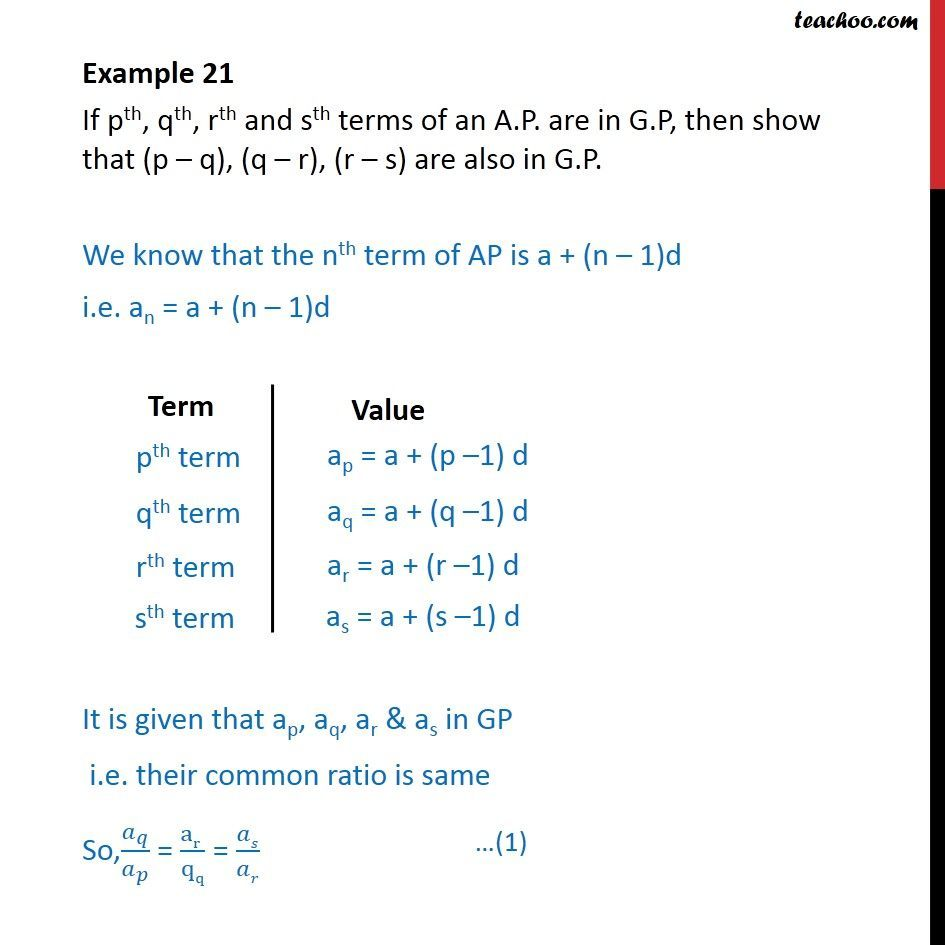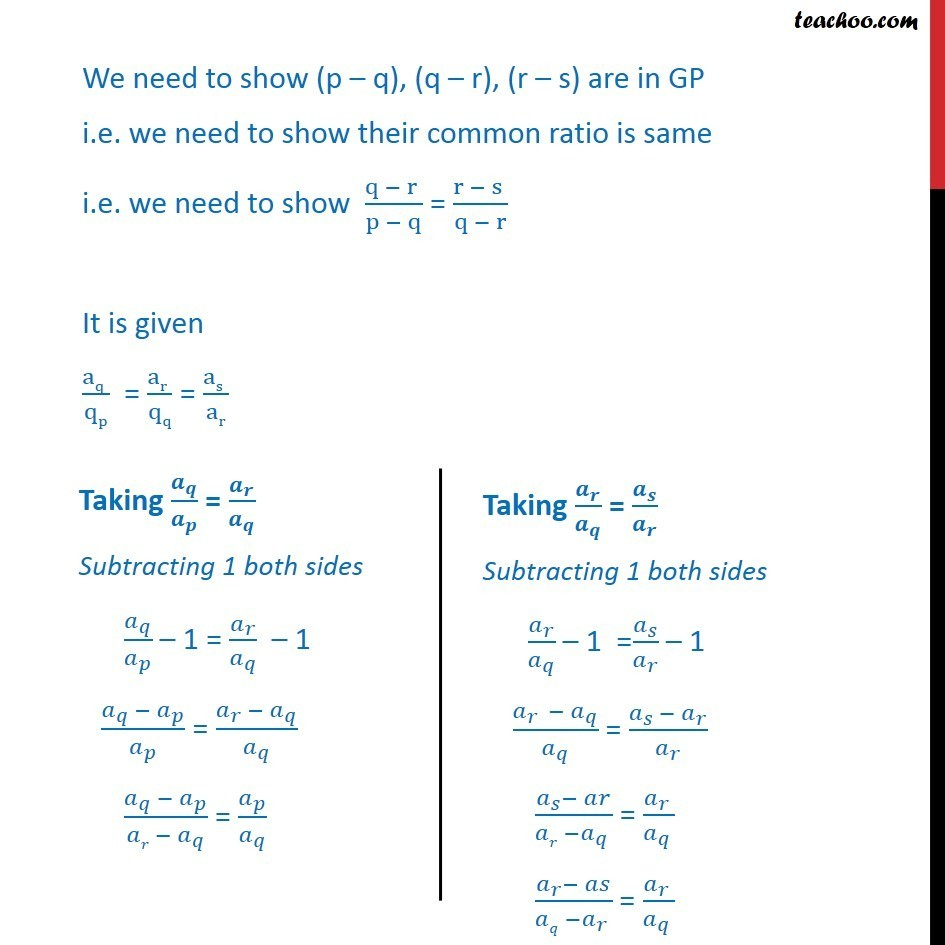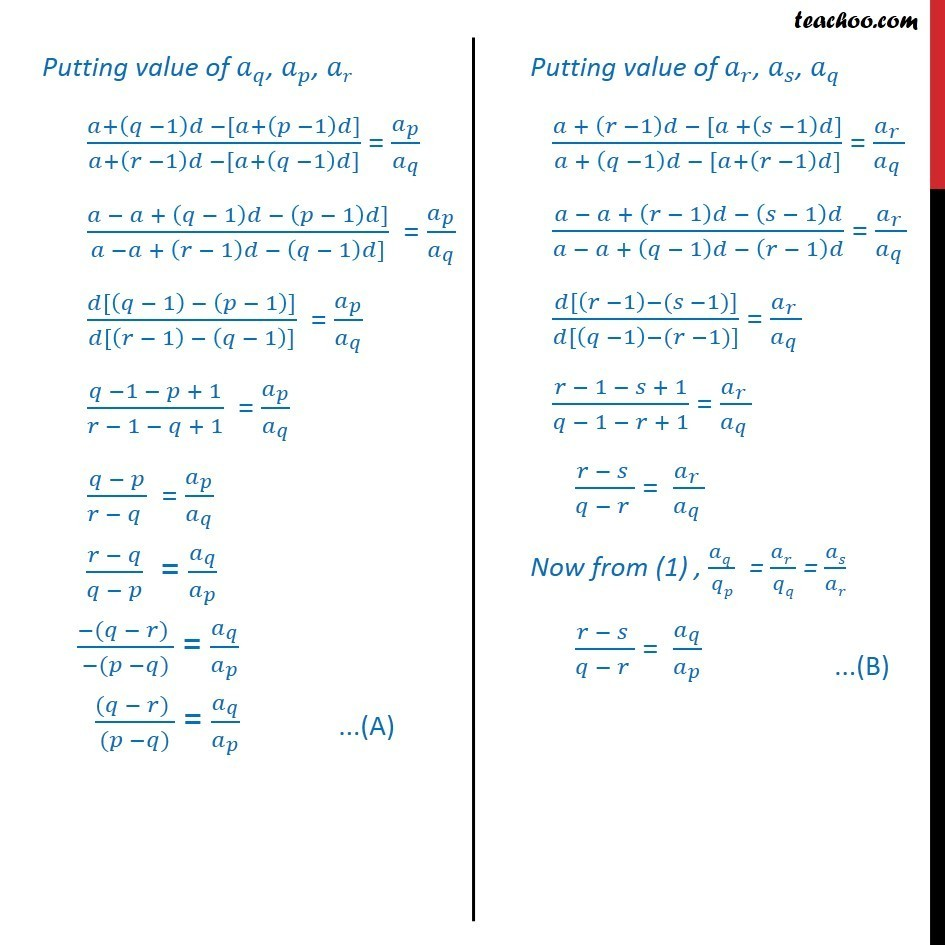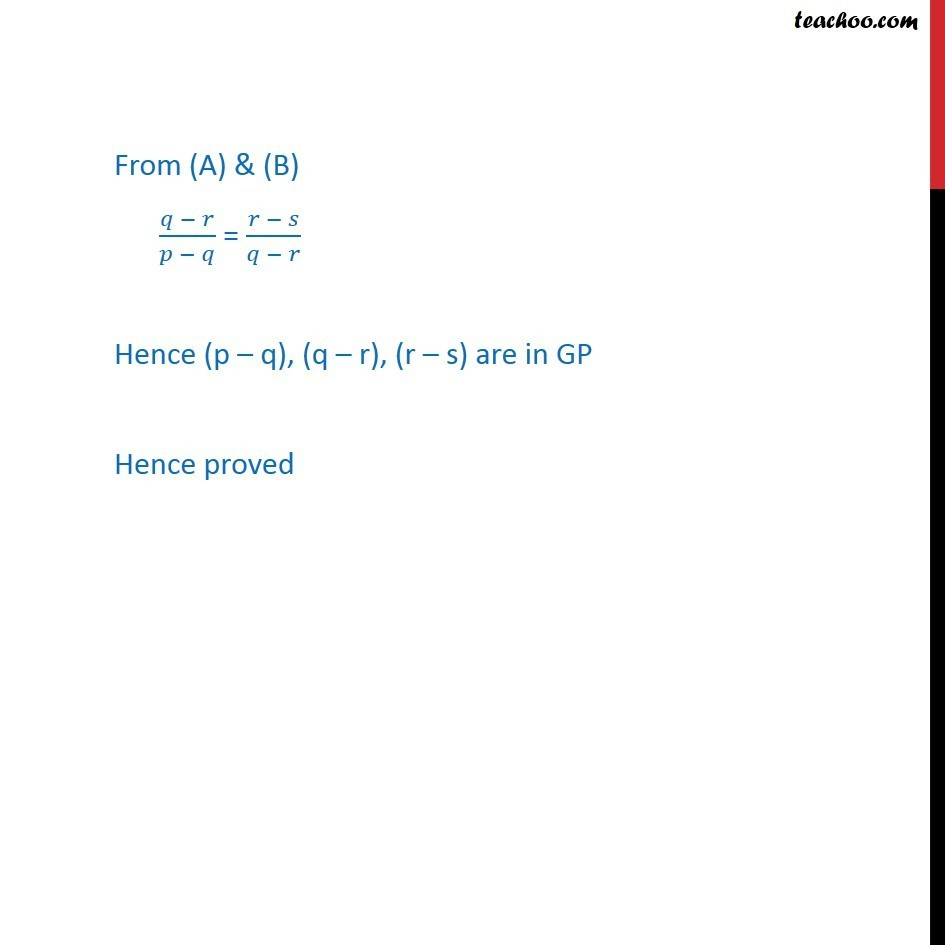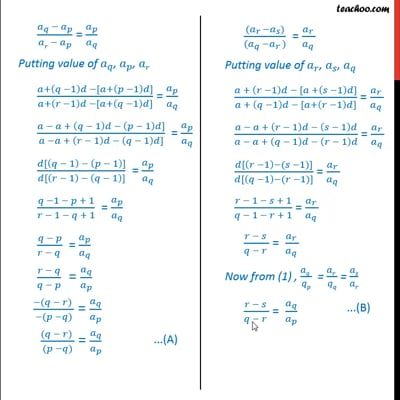This video is only available for Teachoo black users

Learn in your speed, with individual attention - Teachoo Maths 1-on-1 Class

### Transcript

Question 8 If pth, qth, rth and sth terms of an A.P. are in G.P, then show that (p – q), (q – r), (r – s) are also in G.P. We know that the nth term of AP is a + (n – 1)d i.e. an = a + (n – 1)d It is given that ap, aq, ar & as in GP i.e. their common ratio is same So,𝑎_𝑞/𝑎_𝑝 = (ar )/qq = 𝑎𝑠/𝑎𝑟 We need to show (p – q), (q – r), (r – s) are in GP i.e. we need to show their common ratio is same i.e. we need to show (q − r )/(p − q) = (r − s )/(q − r) It is given (aq )/qp = (ar )/qq = (as )/ar From (A) & (B) (𝑞 − 𝑟)/(𝑝 − 𝑞) = (𝑟 − 𝑠)/(𝑞 − 𝑟) Hence (p – q), (q – r), (r – s) are in GP Hence proved